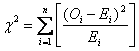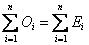# Statistics Assignment Help With Chi Square Test Of Goodness Of Fit

A very powerful test for testing the significance of the discrepancy between theory and experiment was given by Prof. Karl Pearson in 1900 and is known as “Chi-Square test of goodness of fit”. It enables us to find if the deviation of the experiment from theory is just by chance or is it really due to the inadequacy of the theory to fit the observed data.If Oi, (i= 1, 2,…, n) is a set of observed frequencies and Ei, (i= 1, 2,…, n) is the corresponding set of expected frequencies, then Karl Pearson’s chi-square, given byFollows chi-square distribution with (n-1) degrees of freedom.

### Email Based Homework Help in Chi Square Test Of Goodness Of Fit

To submit Chi Square Test Of Goodness Of Fit assignment click here.

### Following are some of the topics in Exact Sampling distributions in which we provide help:

Online Statistics Help | Statistics Math Help | Statistics probability help | Statistics help | College statistics help | Business statistics help | Elementary statistics help | Probability and statistics help | Statistics tutor | Statistic Homework help | Excel help | Mathematics help | Matlab help | MegaStat help | Minitab help | PHStat2 help | POM/QM help | R code and S-Plus help | SAS help | SPSS Help | Stata help | TDISK help | Tree Plan help | Online Tutoring